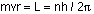## Understanding the atom

8-9-99

### Rutherford scattering

Let's focus on the atom, starting from a historical perspective. Ernest Rutherford did a wonderful experiment in which he fired alpha particles (basically helium nuclei) at a very thin gold foil. He got a rather surprising result: rather than all the particle passing straight through the foil, many were scattered off at large angles, some even coming straight back. This was inconsistent with the plum-pudding model of the atom, in which the atom was viewed as tiny electrons embedded in a dispersed pudding of positive charge. Rutherford proposed that the positive charge must really be localized, concentrated in a small nucleus.

This led to the planetary model of the atom, with electrons orbiting the nucleus like planets orbiting the Sun.

### Line spectra

If the atom looked like a solar system, how could line spectra be explained? Line spectra are what you get when you excite gases with a high voltage. Gases emit light at only a few sharply-defined frequencies, and the frequencies are different for different gases. These emission spectra, then, are made up of a few well-defined lines.

Gases will also selectively absorb light at these same frequencies. You can see this if you expose a gas to a continuous spectrum of light. The absorption spectra will be very similar to a continuous spectrum, except for a few dark lines corresponding to the frequencies absorbed by the gas.

### The Bohr model

The Bohr model is a planetary model of the atom that explains things like line spectra. Neils Bohr proposed that the electrons orbiting the atom could only occupy certain orbits, orbits in which the angular momentum satisfied a particular equation: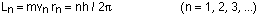where m is the mass of the electron, r is the radius of the orbit, and v is the orbital speed of the electron.

In other words, Bohr was proposing that the angular momentum of an electron in an atom is quantized.

What does quantization of the angular momentum mean for the energy of the electron in a particular orbit? We can analyze the energy very simply using concepts of circular motion and the potential energy associated with two charges. The electron has a charge of -e, while the nucleus has a charge of +Ze, where Z is the atomic number of the element. The energy is then given by: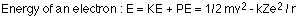The electron is experiencing uniform circular motion, with the only force on it being the attractive force between the negative electron and the positive nucleus. Thus: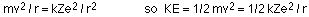Plugging this back into the energy equation gives: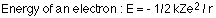If you rearrange the angular momentum equation to solve for the velocity, and then plug that back into the equation:and solve that for r, you get: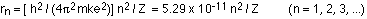This can now be substituted into the energy equation, giving the total energy of the nth level:### Energy level diagrams and the hydrogen atom

It's often helpful to draw a diagram showing the energy levels for the particular element you're interested in. The diagram for hydrogen is shown on page 918 in the text. Hydrogen's easy to deal with because there's only one electron to worry about.

The n = 1 state is known as the ground state, while higher n states are known as excited states. If the electron in the atom makes a transition from a particular state to a lower state, it is losing energy. To conserve energy, a photon with an energy equal to the energy difference between the states will be emitted by the atom. In the hydrogen atom, with Z = 1, the energy of the emitted photon can be found using: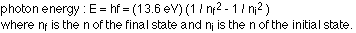Atoms can also absorb photons. If a photon with an energy equal to the energy difference between two levels is incident on an atom, the photon can be absorbed, raising the electron up to the higher level.

### Angular momentum

Bohr's model of the atom was based on the idea the angular momentum is quantized, and quantized in a particular way. de Broglie came up with an explanation for why the angular momentum might be quantized in this way. de Broglie realized that if you use the wavelength associated with the electron, and only allow for standing waves to exist in any orbit (in other words, the circumference of the orbit has to be an integral number of wavelengths), then you arrive at the same relationship for the angular momentum that Bohr got.

The derivation works like this, starting from the idea that the circumference of the circular orbit must be an integral number of wavelengths:Taking the wavelength to be the de Broglie wavelength, this becomes: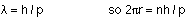The momentum, p, is simply mv as long as we're talking about non-relativistic speeds, so this becomes: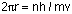Rearranging this a little, and recognizing that the angular momentum for a point mass is simply L = mvr, gives the Bohr relationship: